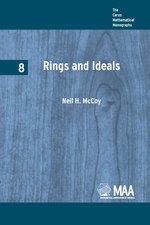Have library access? Log in through your library# Rings and Ideals

NEAL H. McCOY
Volume: 8
Copyright Date: 1948
Edition: 1
Pages: 229
https://www.jstor.org/stable/10.4169/j.ctt5hh950

## Table of Contents

1. Front Matter
(pp. i-vi)
2. PREFACE
(pp. vii-x)
Neal Henry McCoy
3. Table of Contents
(pp. xi-xii)
4. Chapter I DEFINITIONS AND FUNDAMENTAL PROPERTIES
(pp. 1-30)

Let us consider a setRof elements a, b, c, · · · , such that for arbitrary elementsaandbofRthere is a uniquely definedsum a + bandproduct ab(sometimes written asa·b) which are also elements ofR. The wordsadditionandmultiplication, as in the ordinary usage of elementary algebra, will be respectively associated with the operations of forming a sum, or a product, of elements ofR. Such a set is said to be aringif addition and multiplication have the five properties listed below, it being assumed...

5. Chapter II POLYNOMIAL RINGS
(pp. 31-51)

In the preceding chapter, we gave a number of examples of rings and used them to illustrate various points of the theory. We now introduce another important class of rings.

LetRbe an arbitrary ring,xan arbitrary symbol orindeterminate(not an element ofR), and consider all finite expressions of the type

(3)$f(x) = {a_0}{x^0} + {a_1}{x^1} + {a_2}{x^2} + ... + {a_n}{x^n}$,

where${a_i}$, is an element of$R(i = 0,1,.,n)$. Such an expression may be called apolynomialinxwith coefficients inR. It is assumed that the symbolxis commutative with all elements ofR, that is, thataxis not to be...

6. Chapter III IDEALS AND HOMOMORPHISMS
(pp. 52-82)

In accordance with previous use of the term, asubringof a ringRis a setSof elements ofRsuch that, with respect to the addition and multiplication defined inR,Sis itself a ring. Previously mentioned examples of subrings are the subring of all even integers in the ring of all integers, the subring of all integers in the field of rational numbers, and the subring of all finite subsets of$\mathcal{J}$in the ring of all subsets of$\mathcal{J}$.

Although subrings are sometimes of interest, it will be shown presently that subsystems of more...

7. Chapter IV SOME IMBEDDING THEOREMS
(pp. 83-95)

IfRis a given ring, we may sometimes be interested in constructing a ringSwhich containsRas a subring and which has some property not present inR. In this chapter, we shall consider some constructions of this type. However, before proceeding, we shall prove a fundamental theorem which states that it is often sufficient to construct a ring containing a subringisomorphictoR.

First we prove the following almost obvious

Lemma.If S is a ring, and T a set of elements in one-to-one correspondence with the elements of S, then addition and multiplication may...

8. Chapter V PRIME IDEALS IN COMMUTATIVE RINGS
(pp. 96-113)

One of the most important properties of a prime integerpis that a product of two integers is divisible byponly if at least one of the integers is divisible byp. In the notation of principal ideals, this can be restated as follows. Ifpis a prime, then${n_1}{n_2} \equiv 0(p)$implies that${n_1} \equiv 0(p)$or${n_2} \equiv 0(p)$, or both. Now ifRis an arbitrarycommutativering, an idealpinRis said to be aprime idealif and only if$ab \equiv 0(p)$implies that$a \equiv b \equiv 0(p)$, or both. Thus the prime ideals inIare precisely the ideals...

9. Chapter VI DIRECT AND SUBDIRECT SUMS
(pp. 114-137)

Let${S_1}$and${S_2}$be any two rings, and consider the set of all symbols$({S_1},{S_2})$where${s_1} \in {S_1},{s_2} \in {S_2}$. If we define addition and multiplication of these symbols by

$({s_1},{s_2}) + ({t_1},{t_2}) = ({s_1} + {t_1},{s_2} + {t_2})$,

and$({s_1},{s_2})({t_1},{t_2}) = ({s_1}{t_1},{s_2}{t_2})$,

it is easy to verify that this set becomes a ringSwhich we call thedirect sumof${S_1}$and${S_2}$, and denote by${S_1}\dot + {S_2}$.

Clearly, the zero element of$S = {S_1}\dot + {S_2}$is (0, 0), the first 0 being the zero of${S_1}$, the second the zero of${S_2}$. If${S_1}$and${S_2}$have unit elements${e_1}$and${e_2}$respectively, thenShas the unit element$({e_1},{e_2})$. If both${S_1}$...

10. Chapter VII BOOLEAN RINGS AND SOME GENERALIZATIONS
(pp. 138-149)

The so-called “algebra of logic” had its origin in the fundamental work of the English mathematician and logician, George Boole (1815–1864), and is therefore usually calledBoolean algebra. Although there is a very extensive literature on this subject, we shall present just enough to furnish a partial setting for the material of the next section.

Let us consider a set of “statements” which may be either true or false. For concreteness, we shall consider the class$\mathcal{C}$of all books in the Library of Congress, and statements about any bookxof$\mathcal{C}$. As examples, we may use the...

11. Chapter VIII RINGS OF MATRICES
(pp. 150-179)

In §2 of Chapter I, we introduced, as an illustration of the concept of ring, the ring of all real matrices of order 2, and suggested that this example could be generalized in at least two different ways. In the present chapter we shall make these generalizations and study matrices in some detail. The necessary definitions will be given presently, but first we make a few remarks which will help to put the rest of the chapter in proper perspective.

The importance of the theory of matrices stems from at least two facts. In the first place, the theory furnishes...

12. Chapter IX FURTHER THEORY OF IDEALS IN COMMUTATIVE RINGS
(pp. 180-209)

Throughout this chapter we shall considercommutativerings only.

It was pointed out in Chapter V that in some important respects the prime ideals in an arbitrary commutative ring play a role similar to that of the primes in the ringIof integers. We now study a more general class of ideals which will be seen to bear roughly the same relation to thepowersof a prime integer that the prime ideals do to the primes themselves.

An ideal q in the commutative ringRis said to beprimaryif$ab \equiv 0(q)$,$a \ne 0(q)$, imply that${b^i} \equiv 0(q)$for some...

13. BIBLIOGRAPHY
(pp. 210-214)
14. INDEX OF TERMS
(pp. 215-216)